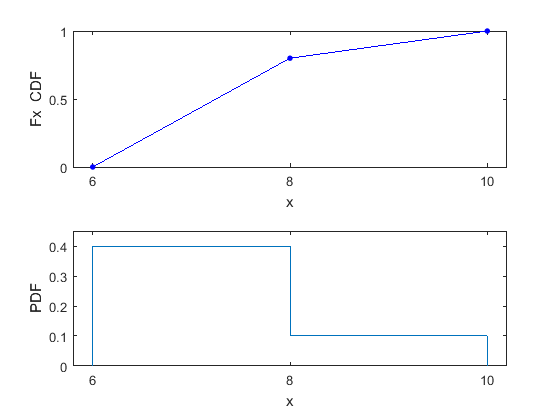# makedist

Create probability distribution object

## Syntax

``pd = makedist(distname)``
``pd = makedist(distname,Name,Value)``
``list = makedist``

## Description

example

````pd = makedist(distname)` creates a probability distribution object for the distribution `distname`, using the default parameter values.Use `makedist` to specify uniform, normal, multinomial, piecewise linear, or triangular distribution objects. If you have Statistics and Machine Learning Toolbox™ software, you can use `makedist` to create objects for other distributions, such as the Gamma or Weibull distributions. For more information, see `makedist` (Statistics and Machine Learning Toolbox).To truncate the probability distribution to a specified interval, use `truncate` (Statistics and Machine Learning Toolbox).```

example

````pd = makedist(distname,Name,Value)` creates a probability distribution object with one or more distribution parameter values specified by name-value pair arguments.```
````list = makedist` returns a cell array `list` containing a list of the probability distributions that `makedist` can create.```

## Examples

collapse all

Create a normal distribution object using default parameter values.

`pd = makedist('Normal')`
```pd = NormalDistribution Normal distribution mu = 0 sigma = 1 ```

Create a normal distribution object with a mean value of `mu = 75`, and a standard deviation of `sigma = 10`.

`pd = makedist('Normal','mu',75,'sigma',10);`

Create a piecewise linear distribution object for a distribution with values from 6 to 10, where values from 6 to 8 are four times more likely than values from 8 to 10.

`pd = makedist('PieceWiselinear','x',[6 8 10],'Fx',[0 0.8 1]);`

You specify the cumulative distribution function `Fx` as [0 0.8 1] because the difference between 0.8 and 0 is four times the difference between 1 and 0.8. As a result, the generated distribution has four times more values between 6 to 8 than between 8 to 10.

The plot shows the specified cumulative distribution function (CDF) and the corresponding probability distribution function (PDF).The piecewise linear CDF corresponds to a piecewise constant PDF.

## Input Arguments

collapse all

Distribution name, specified as one of the following values:

Distribution NameDescription
`'Uniform'`Uniform distribution — You specify the lower and upper bounds of the distribution.
`'Normal'`Normal distribution — You specify the mean and standard deviation of the distribution.
`'Multinomial'`Multinomial distribution — In a multinomial distribution, the outcome is one of `1`, `2`, ..., `k`. You specify the probability of each outcome, [p1,p2,..., pk]. The probabilities should sum to `1`.
`'PiecewiseLinear'`

Piecewise Linear distribution — Use this distribution to make a custom distribution by specifying a piecewise linear cumulative distribution function (CDF). You specify the vector of values, `x`, at which the CDF changes slope and the corresponding vector of CDF values, `Fx`. The `Fx` value at any `x` is the probability of getting a value less than or equal to `x`. A piecewise linear CDF is created by linearly connecting the known CDF values, `Fx`. This piecewise linear CDF corresponds to a piecewise constant probability distribution function.

For an example, see Specify Piecewise Linear Distribution Object.

`'Triangular'`Triangular distribution — You specify the lower limit, peak location, and upper limit of the distribution.

For information about these distributions, see the Probability Distributions (Statistics and Machine Learning Toolbox) category.

Note

If you have Statistics and Machine Learning Toolbox software, you can use `makedist` to create objects for other distributions, such as the Gamma or Weibull distributions. For more information, see `makedist` (Statistics and Machine Learning Toolbox).

### Name-Value Pair Arguments

Specify optional comma-separated pairs of `Name,Value` arguments. `Name` is the argument name and `Value` is the corresponding value. `Name` must appear inside quotes. You can specify several name and value pair arguments in any order as `Name1,Value1,...,NameN,ValueN`.

Example: `makedist('Normal','mu',10)` specifies a normal distribution with parameter `mu` equal to 10, and parameter `sigma` equal to the default value of 1.
Multinomial Distribution

collapse all

Outcome probabilities, specified as a vector of scalar values in the range [0,1]. The probabilities sum to 1 and correspond to outcomes [1, 2, ..., k], where k is the number of elements in the probabilities vector.

Example: `'probabilities',[0.1 0.2 0.5 0.2]` gives the probabilities that the outcome is 1, 2, 3, or 4, respectively.

Data Types: `single` | `double`

Normal Distribution

collapse all

Example: `'mu',2`

Data Types: `single` | `double`

Example: `'sigma',2`

Data Types: `single` | `double`

Piecewise Linear Distribution

collapse all

Example: `'x',[1 2 3]`

Data Types: `single` | `double`

Example: `'Fx',[0.2 0.5 1]`

Data Types: `single` | `double`

Triangular Distribution

collapse all

Example: `'a',-2`

Data Types: `single` | `double`

Example: `'b',1`

Data Types: `single` | `double`

Example: `'c',5`

Data Types: `single` | `double`

Uniform Distribution

collapse all

Example: `'lower',-4`

Data Types: `single` | `double`

Example: `'upper',2`

Data Types: `single` | `double`

## Output Arguments

collapse all

Probability distribution, returned as a probability distribution object of the type specified by `distname`.

List of probability distributions that `makedist` can create, returned as a cell array of character vectors.

| (Statistics and Machine Learning Toolbox)

Introduced in R2014a